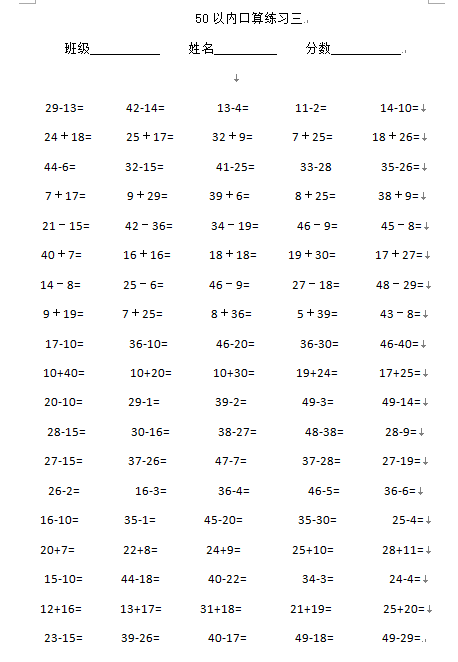50以内口算练习三

29-13=          42-14=            13-4=          11-2=           14-10=
24＋18=          25＋17=          32＋9=         7＋25=         18＋26=
44-6=           32-15=            41-25=           33-28           35-26=

【DOC文档10页】小学一年级数学下册50以内加减法练习题 A4电子版资料_可直接打印_会员免费下载##### 评论信息